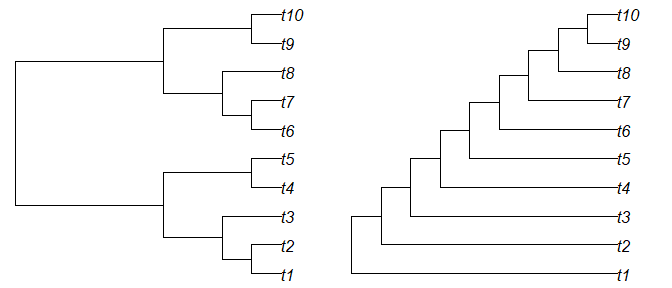# Using TBRDist

#### 2020-09-17

‘TBRDist’ is an R package to compare unrooted phylogenetic trees using the SPR, TBR and Replug distances.

This document assumes familiarity with the process of loading trees into R.

We’ll work with some simple trees generated using the ‘TreeTools’ package.

library('TreeTools', quietly = TRUE, warn.conflicts = FALSE)

tree1 <- BalancedTree(10)
tree2 <- PectinateTree(10)

origPar <- par(mfrow = 1:2, mar = rep(0.2, 4)) # Set up plotting area
plot(tree1)
plot(tree2)## Calculating distances

After installing TBRDist (install.packages('TBRDist')), load the package into R with

library('TBRDist')

To generate an approximate SPR distance between two unrooted trees, use:

USPRDist(tree1, tree2)
##  2

For Replug distances, it’s:

ReplugDist(tree1, tree2)
##  2

For TBR distances, use:

TBRDist(tree1, tree2, exact = TRUE)
##  1

When calculating an exact TBR distance, we receive information on the maximum agreement forest for free (i.e. with no extra processing cost):

TBRDist(tree1, tree2, exact = TRUE, maf = TRUE)
## $tbr_exact ##  1 ## ##$maf_1
##  "(t4,t5,((t1,t2),t3)); ((t9,t10),t8,(t7,t6));"
##
## $maf_2 ##  "(((t1,t2),t3),t4,t5); ((t6,t7),(t9,t10),t8);" Once trees have more than about a dozen tips, it becomes slow to calculate the exact distance. In the interests of speed, we may wish to approximate the value of the TBR distance: TBRDist(tree1, tree2, exact = FALSE) ##$tbr_min
##  1
##
## $tbr_max ##  3 ## Comparing multiple trees To avoid multiple calls, each function can also be used to compare lists of trees (or multiPhylo objects) against a single tree: TBRDist(tree1, list(tree1, tree2), exact = TRUE, maf = TRUE) ##$tbr_exact
##  0 1
##
## $maf_1 ##  "((((t1,t2),t3),(t4,t5)),(t9,t10),((t6,t7),t8));" ##  "(t4,t5,((t1,t2),t3)); ((t9,t10),t8,(t7,t6));" ## ##$maf_2
##  "((((t1,t2),t3),(t4,t5)),(t9,t10),((t6,t7),t8));"
##  "(((t1,t2),t3),t4,t5); ((t6,t7),(t9,t10),t8);"

Or against each corresponding entry in a second list:

USPRDist(list(tree1, tree2, tree2), list(tree2, tree1, tree2))
##  2 2 0

## Citation

If you use ‘TBRDist’ in your research, please cite:

• Chris Whidden and Frederick A. Matsen IV (2017). Calculating the Unrooted Subtree-Prune-and-Regraft Distance. eprint arXiv:1511.07529.

Optionally, a citation to the ‘TBRDist’ R package would be welcome: